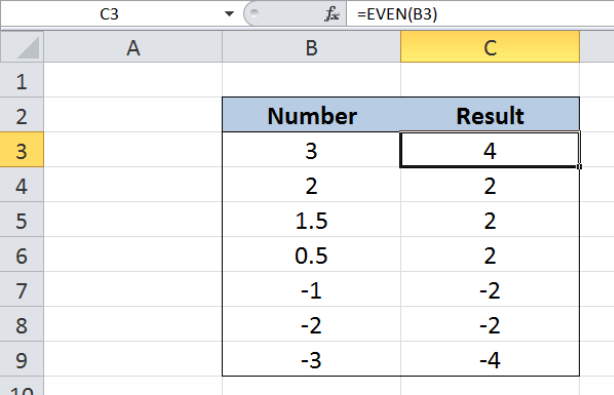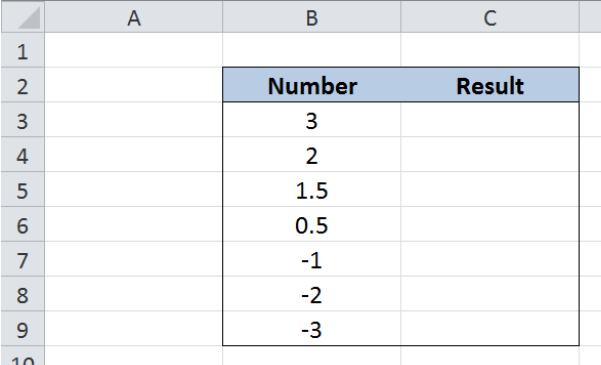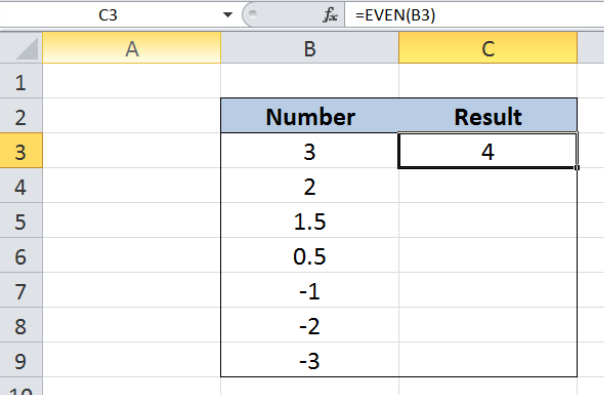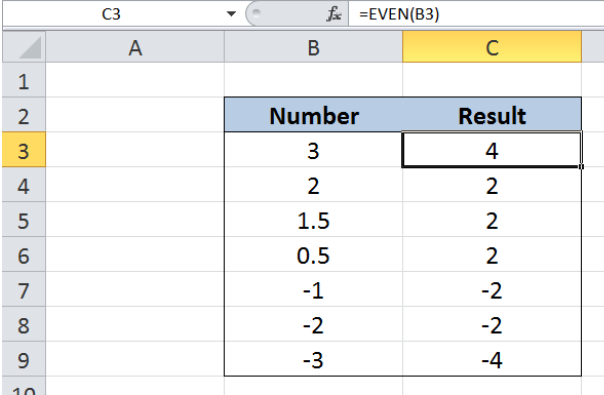Get instant live expert help with Excel or Google Sheets“My Excelchat expert helped me in less than 20 minutes, saving me what would have been 5 hours of work!”

#### Post your problem and you’ll get Expert help in seconds.

Your message must be at least 40 characters
Our professional Expert are available now. Your privacy is guaranteed.

# Excel EVEN Function

EVEN is a function in Excel that rounds up a number to the nearest even integer.  This step by step tutorial will assist all levels of Excel users in using the EVEN function.Figure 1. Final result: Excel EVEN function

## Syntax of the EVEN Function

`=EVEN(number)`

• number – the value we want to round
• If number is an even integer, no rounding occurs
• EVEN function returns the #VALUE! error if the argument number is not a numeric value

## Setting up our Data

Our data consists of two columns : Number (column B) and Result (column C).  Column B contains the numbers we want to round using the EVEN function. The results will be recorded in column C.Figure 2. Sample data for the EVEN function

## Round up to nearest even integer using EVEN function

We want to round up the numbers to the nearest even integer using the EVEN function.  Let us follow these steps:

Step 1.  Select cell C3

Step 2.  Enter the formula: `=``EVEN(B3) `

Step 3.  Press ENTER

Step 4:  Copy the formula in cell C3 to cells C4:C9 by clicking the “+” icon at the bottom-right corner of cell C3 and dragging it downFigure 3. Entering the formula for the EVEN function

As shown in cell C3, our formula rounds up the value “3” to the nearest even integer, which is “4”.

Below table shows all numbers in column B being rounded up to the nearest even integer by the EVEN function.

For numbers 2 and -2, no rounding occurs because these numbers are already even.  For the numbers 0.5 and 1.5, both are rounded up to the next even integer “2”, skipping the integer “1” because “1” is not even.  For the numbers -1 and -3, these numbers are rounded to -2 and -4, respectively.Figure 4. Output:  Rounding up to nearest even integer using EVEN function

Note that the EVEN function always rounds a number away from zero.  Hence, a positive number becomes more positive or larger in value, while a negative number also becomes more negative, or smaller in value.

## Instant Connection to an Expert through our Excelchat Service

Most of the time, the problem you will need to solve will be more complex than a simple application of a formula or function. If you want to save hours of research and frustration, try our live Excelchat service! Our Excel Experts are available 24/7 to answer any Excel question you may have. We guarantee a connection within 30 seconds and a customized solution within 20 minutes.

### Did this post not answer your question? Get a solution from connecting with the expert.Another blog reader asked this question today on Excelchat:
Solution examplesI need a formula that has these 2 rules. If letter was rec'd between 1st and 15th = 1st of the month following letter rec'd date. If letter was rec'd between 16thst and end of the month= 1st of the 2nd month following letter rec'd date.
Solved by V. F. in 20 minsI need a column to total (Hours)(85) + (Travel)(.55) per line
Solved by F. Q. in 20 minsTrying to figure out what is wrong with my formula in Oct-ZD tab in column AY and BG.
Solved by O. Q. in 8 minsSo I have used vlookup to find the price of a product on a different sheet and then I have tried to times that by a quantity. e.g. =vlookup(d4,table35,2,false)*e4 This is the formula I typed in which worked for another list I did but for some reason it's not working now even though everything is practically the same, apart from the contents. Could you help?
Solved by B. U. in 60 minsI have a column containing date and time data together and i need to isolate the time data. When I tried to use text to column, it changed all the times to AM. There is an extremely large amount of data - over 550,000 rows so manually changing it won't work. The file is too large to attach.
Solved by S. E. in 40 mins## Subscribe to Excelchat.coAnother blog reader asked this question today on Excelchat: